For signal traces, a via is a structure in a PCB or package substrate that allows for the transfer of signals in a vertical manner from one layer to another. A typical via structure used for differential signals is depicted in Figure 1. The structure consists of two signal vias and two return path vias that are connected in the following order: Return via – Signal P – Signal N – Return via – where the return vias are usually connected to GND planes.

We mark this via structure as G-S-S-G. However, can this common via structure transfer any data rate or is it limited and have a defined bandwidth? If so, what limits its bandwidth? And what can be done when the bandwidth of the signal is greater than the bandwidth of the G-S-S-G structure?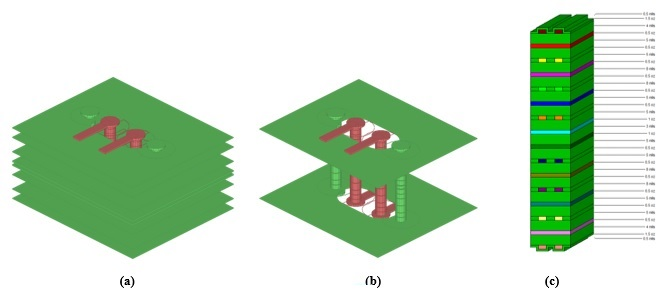Figure 1: G-S-S-G via structure: (a) with all planes in the stackup (b) display only with the external planes (c) 16 layers stackup

The signal vias depicted in Figure 1 pass from layer 1 (top) to layer 16 (bottom) in a stackup structure with 16 layers: see Figure 1(c). The differential impedance of the input and output traces are 90 ohms and the traces' length is 50 mil. The dimensions of the via structure (drills, anti-pad size and shape) have been designed for a via impedance of approximately 90 Ohms for a 40 mil signal vias pitch. The differential port impedance for the S parameters is also 90 Ohms.

We will examine the via described in Figure 1 in two different cases: (1) a PCB with high loss materials that has copper surface roughness Rz= 0.4 mil (10 μm) and a dissipation factor Df = 0.025 (2) a PCB with low loss materials that has Rz=0.08 mil (2 μm) and Df = 0.002. First, we will write the power balance equation for a differential signal entering port 1 whose power is |VD1|2 / 2ZD: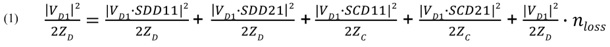Where VD1, ZD and ZC are the differential signal amplitude, the differential impedance and the common impedance, respectively. |SDD11|2 and |SDD21|2 are the percentage of the power that reflects back to port 1 or transfers to port 2, respectively. |SCD11|2 and |SCD21|2 are the percentage of the power that is converted to a common signal and reflects back to port 1 or transfers to port 2, respectively. nloss is the percentage of the power that is dissipated by losses. Due to the high symmetry in the simulation between the P line and N line, the mode conversion (SCD) is very low, so we will write (1) as an approximation: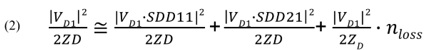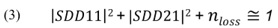The total losses in the system consist of: (1) conductor loss (including surface roughness) (2) dielectric loss (3) radiation loss into the cavity. When the signal passes in the via and current flows in it, according to Maxwell’s equations, the via will radiate an electromagnetic wave that will propagate radially between the planes in the layers in which the via passes. We will write the components of the total losses in equation (4):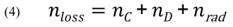where nC, nD and nrad describe the percentage of the input power that is lost as a result of conductor loss, dielectric loss and radiation loss into the cavity, respectively.

The task now is to isolate the radiation loss nrad and investigate the frequency from which the via structure G-S-S-G starts to radiate significantly into the PCB. In order to do this, we will examine the via structure described in Figure 1 in a model with lossless materials too. To do this, we change all the metals from copper to a perfect electric conductor (PEC) and eliminate the surface roughness in order to zero out the conductor loss (nC = 0 ). Then, we set Df=0  in order to zero out the dielectric loss (nD = 0). Next, we define the simulation model’s boundaries to be of absorbing boundaries type.

In this case, the electromagnetic fields that the vias radiate into the PCB and reach the model’s boundaries will be absorbed by the model’s boundaries and will not be reflected to propagate in the PCB. In this case, we will get from (4) that in a model with lossless materials nloss = nrad  and (3) becomes: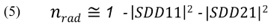This means that from (5) in a model with lossless materials, one may calculate radiation loss given the S parameters. The calculations of  nloss from the simulations’ S parameter results in a model with high loss materials and in a model with low loss materials, and of nrad  in a model with lossless materials appear in Figure 2.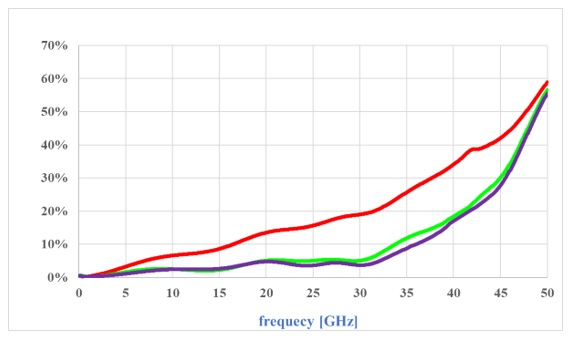Figure 2: nloss : Red – high loss materials, Green – low loss materials (calculated with (3))

Purple: nrad - lossless materials (calculated with (5))

In Figure 2, the purple line describes the radiation loss by frequency in the model with lossless materials. It may be seen that up to a frequency of 30 GHz, the radiation losses in the G-S-S-G via structure are quite low (<5%).

Above 30 GHz, the radiation losses begin to increase substantially. Radiation of vias into the cavity cause a number of very significant signal and power integrity (SIPI) problems: (1) a significant increase in differential insertion loss of the signal, with all resulting implications (2) an increase of cross talk between signals (3) increase of the ISI because in a real PCB, the radiation is reflected from the PCB boundaries (4) increased ground and power supplies noise (5) increase in jitter of signals transmitted from components that are connected to these supplies.

Because the radiation from vias has such an extensive, significant effect on SIPI, we will not want to work with vias at frequencies at which their radiation is high. To this end we will define the radiation cutoff frequency fC.R of a via structure as “a frequency at which the radiation losses of the vias begin to increase significantly.”

In Figure 2, in the example of the G-S-S-G via structure, we see that fC.R is approximately 30 GHz. At a frequency of 50 GHz, for example, more than half (55%) of the power of the differential signal is lost as a result of radiation of the vias to the PCB. The green line depicts the percentage of the power that is dissipated as a result of the losses by frequency, using the model with low loss materials (as detailed above).

One cannot know from the graph how the losses are divided between conductor loss, dielectric loss and radiation loss, but one may clearly see that most losses are radiation losses. This result makes sense because the lengths of the traces and the vias in the model are very short, so the conductor loss is low, and because the dissipation factor is very small, the dielectric loss is low, meaning that most loss is radiation loss.

This is the place to state that when we completely zero out the losses in the model, the model is very sensitive to resonances that might be developed in it because there is nothing to damp the resonances. This will manifest in non-smooth S parameter results with sharp peaks and dips. Therefore, in practical terms, it is still worth adding some losses to the model even when we are examining radiation losses.

The red line in Figure 2 describes the percentage of the power that dissipates as a result of the losses by frequency when the model is with high loss materials. Here too, one cannot know from the graph exactly what percentage is wasted for each loss. One may see that up to a frequency of 30 GHz, the losses increase at a fixed rate of 0.667 %/GHz, and had significant radiation from the vias not started, this rate would have been kept constant. Because the vias start to radiate significantly from 30 GHz, the loss rate increases substantially.

After showing a method for simulation and calculation of radiation losses from a via structure and the extensive, significant effect that this radiation has on SIPI, we will now examine the two main factors affecting radiation from a via structure: (1) coupling between the signal vias (2) the quantity and location of the return vias.

In order to examine the effect of the coupling between the signal vias, we will use the via structure in Figure 1, but this time without return vias. We examine five different distances between the signal vias (center to center): 26, 32, 40, 50 and 60 mil (see Figure 3). For practical purposes, the minimum distance between the signal vias depends on the diameter of the external pads and the minimum possible distance between them. 32 mil (0.8 mm) and 40 mil (1 mm) are typical pitch sizes for BGA. 50 mil (1.25 mil) and 60 mil (1.5 mm) are typical pitch sizes for high-speed press-fit type connectors.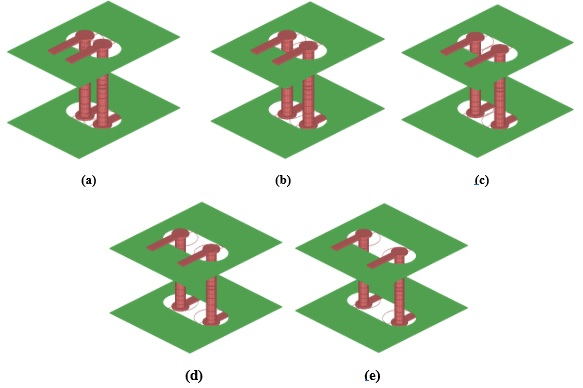Figure 3: via structures with different signal vias pitch: (a) 26 mil (b) 32 mil (c) 40 mil (d) 50 mil (e) 60 mil

Note: for the sake of convenience of viewing, only the external planes of the stackup are shown

The calculations of nrad from the simulations’ S parameter results of the five via structures from Figure 3 (with lossless materials) are shown in Figure 4.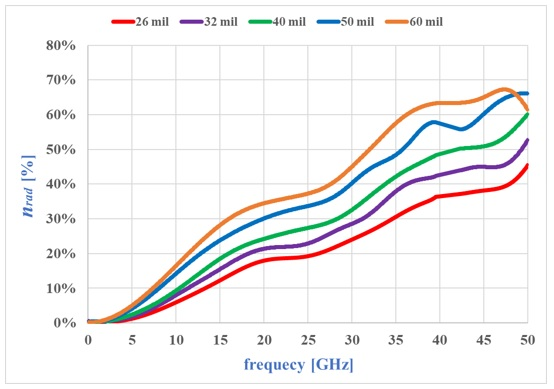Figure 4: nrad of 5 different signal vias pitch: 26, 32, 40, 50 and 60 mil

When a differential signal passes through the signal vias, the currents in the vias flow in opposite directions, so according to the right-hand rule, one via will radiate radially clockwise and the other will radiate radially counterclockwise.

When the signal is purely differential, the magnitude of the currents in the two vias is identical and the same applies to the magnitude of the electromagnetic fields that each via radiates. In this state, the electromagnetic fields that the vias radiate interfere destructively to a certain extent.

Therefore, as we can see in Figure 4: (1) the greater the physical distance between the signal vias, the less good the destructive interference is and the greater the radiation losses are (2) the greater the frequency, the greater the electrical length between the vias, the less good the destructive interference is, and again, the greater the radiation losses become.

As we know, pure differential signals do not exist, and any practical “differential signal” also contains a certain part of a common signal. When a common signal passes in the signal vias, the currents flow in the vias in the same direction and have an identical amplitude. In this state, the electromagnetic fields that the vias radiate interfere constructively and the radiation losses might be greater than in the case of a pure differential signal. Therefore, to restrict the propagation of the fields in the PCB and reduce the radiation losses of the differential vias, return vias are added.

To examine the effect of the quantity of return vias on the radiation losses, we use the via structure in Figure 1, with the signal vias pitch fixed at 40 mil. We next examine four structures with a different number of return vias: 0, 2, 6, and 8 as depicted in Figure 5.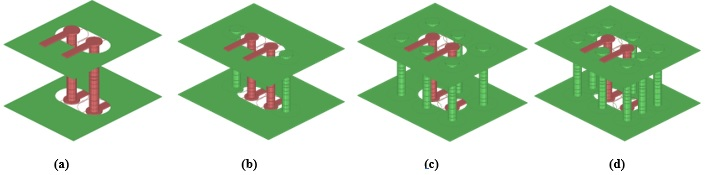Figure 5: Differential via structures with: (a) 0 (b) 2 (c) 6 and (d) 8 return vias

Note: for the sake of convenience of viewing, only the external planes of the stackup are shown

The calculations of nrad from the simulations’ S parameter results of the four via structures from Figure 5 (with lossless materials) are shown in Figure 6.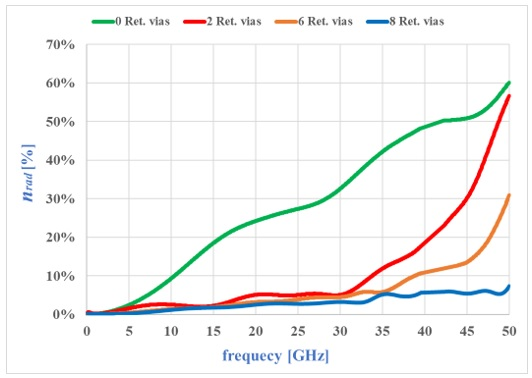Figure 6: nrad of differential via structures with:  0, 2, 6 and 8 return vias

The addition of return vias around the signal vias is a kind of addition of shielding to the structure and contributes to reducing of radiation into the PCB. It should be noted that to reduce the radiation losses, we will have to take care to position the return vias around the signal vias in order to form effective shielding. Of course, should we wish to maintain low radiation losses at high frequencies (shorter wavelengths), we will have to add more return vias and maintain a smaller distance between them of not more than 1/10 of the wavelength.

If we use the definition for a radiation cutoff frequency fC.R of a differential via structure, it may be seen in Figure 6 that in a structure without any return vias, the radiation losses begin to increase significantly starting from 3 GHz, so this is the radiation cutoff frequency of this via structure. Addition of 2 and 6 return vias is able to maintain low radiation losses and increase the radiation cutoff frequency of the structures to 30 GHz and 45 GHz, respectively. Addition of 8 return vias is already able to maintain low radiation losses up to a frequency of at least 50 GHz.

In conclusion, the use of a differential via structure beyond its radiation cutoff frequency fC.R will result in significant radiation into the cavity and many SIPI problems. The radiation losses will result in a decrease in SDD21, but this will not necessarily happen at fC.R frequency because SDD21 is also affected by the amount of reflections, mode conversion, and the other losses. Therefore, it is not always possible to find fC.R directly from SDD21.

Of course, at the end, the overall geometry of the via structure will determine the via impedance, the return loss, insertion loss, and the radiation into the cavity, but one may still see here, albeit roughly, the connection between the number of return vias and the radiation cutoff frequency of the differential via. As we know, the first role of return vias in a differential via structure is to apply a return path with low AC impedance for the return current of the common signal that exists in a practical differential signal. We now also see their second role, which is to reduce the radiation into the cavity of differential signal passes the vias and minimize the resulting SIPI problems, thus allowing the use of differential vias at higher frequencies.